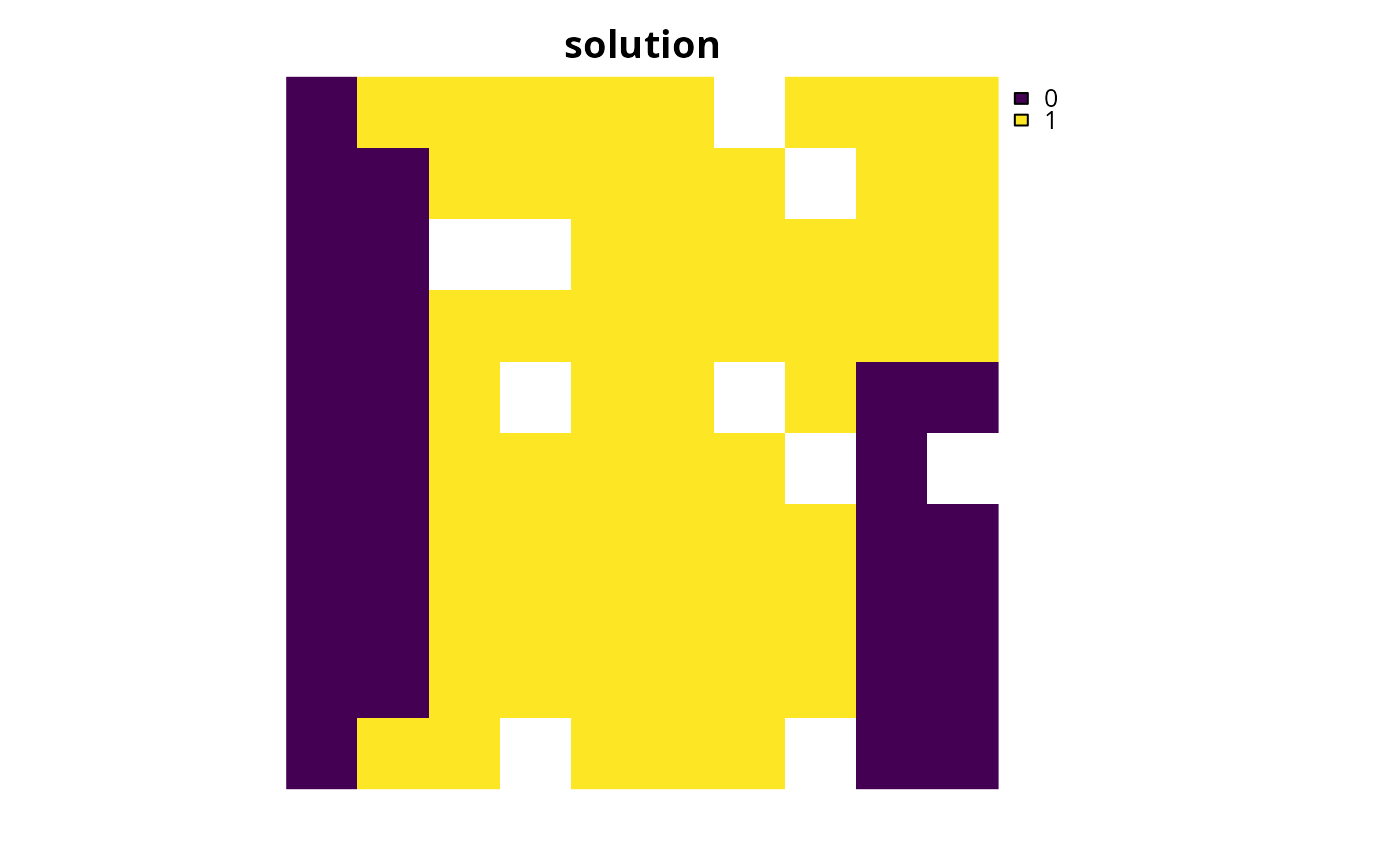Set targets for a conservation planning problem() by manually specifying all the required information for each target. This function is useful because it can be used to customize all aspects of a target. For most cases, targets can be specified using the add_absolute_targets() and add_relative_targets() functions. However, this function can be used to (i) mix absolute and relative targets for different features and zones, (ii) set targets that pertain to the allocations of planning units in multiple zones, and (iii) set targets that require different senses (e.g., targets which specify the solution should not exceed a certain quantity using "<=" values).

add_manual_targets(x, targets)

# S4 method for ConservationProblem,data.frame

# S4 method for ConservationProblem,tbl_df
add_manual_targets(x, targets)

## Arguments

x

problem() (i.e., ConservationProblem) object.

targets

data.frame or tibble::tibble() object. See the Target data format section for more information.

## Value

Object (i.e., ConservationProblem) with the targets added to it.

## Details

Targets are used to specify the minimum amount or proportion of a feature's distribution that needs to be protected. Most conservation planning problems require targets with the exception of the maximum cover (see add_max_cover_objective()) and maximum utility (see add_max_utility_objective()) problems. Attempting to solve problems with objectives that require targets without specifying targets will throw an error.

For problems associated with multiple management zones, this function can be used to set targets that each pertain to a single feature and a single zone. To set targets which can be met through allocating different planning units to multiple zones, see the add_manual_targets() function. An example of a target that could be met through allocations to multiple zones might be where each management zone is expected to result in a different amount of a feature and the target requires that the total amount of the feature in all zones must exceed a certain threshold. In other words, the target does not require that any single zone secure a specific amount of the feature, but the total amount held in all zones must secure a specific amount. Thus the target could, potentially, be met through allocating all planning units to any specific management zone, or through allocating the planning units to different combinations of management zones.

## Targets format

The targets argument should be a data.frame with the following fields (columns):

feature

character name of features in argument to x.

zone

character name of zones in argument to x. This field (column) is optional for arguments to x that do not contain multiple zones.

type

character describing the type of target. Acceptable values include "absolute" and "relative". These values correspond to add_absolute_targets(), and add_relative_targets() respectively.

sense

character sense of the target. Acceptable values include: ">=", "<=", and "=". This field (column) is optional and if it is missing then target senses will default to ">=" values.

target

numeric target threshold.

See targets for an overview of all functions for adding targets.

Other targets: add_absolute_targets(), add_loglinear_targets(), add_relative_targets()

## Examples

# set seed for reproducibility
set.seed(500)

data(sim_pu_raster, sim_features, sim_pu_zones_stack, sim_features_zones)

# create problem with 10% relative targets
p1 <- problem(sim_pu_raster, sim_features) %>%
# \dontrun{
# solve problem
s1 <- solve(p1)

# plot solution
plot(s1, main = "solution", axes = FALSE, box = FALSE)
# }
# create equivalent problem using add_manual_targets
p2 <- problem(sim_pu_raster, sim_features) %>%
type = "relative", sense = ">=",
target = 0.1)) %>%
# \dontrun{
# solve problem
s2 <- solve(p2)

# plot solution
plot(s2, main = "solution", axes = FALSE, box = FALSE)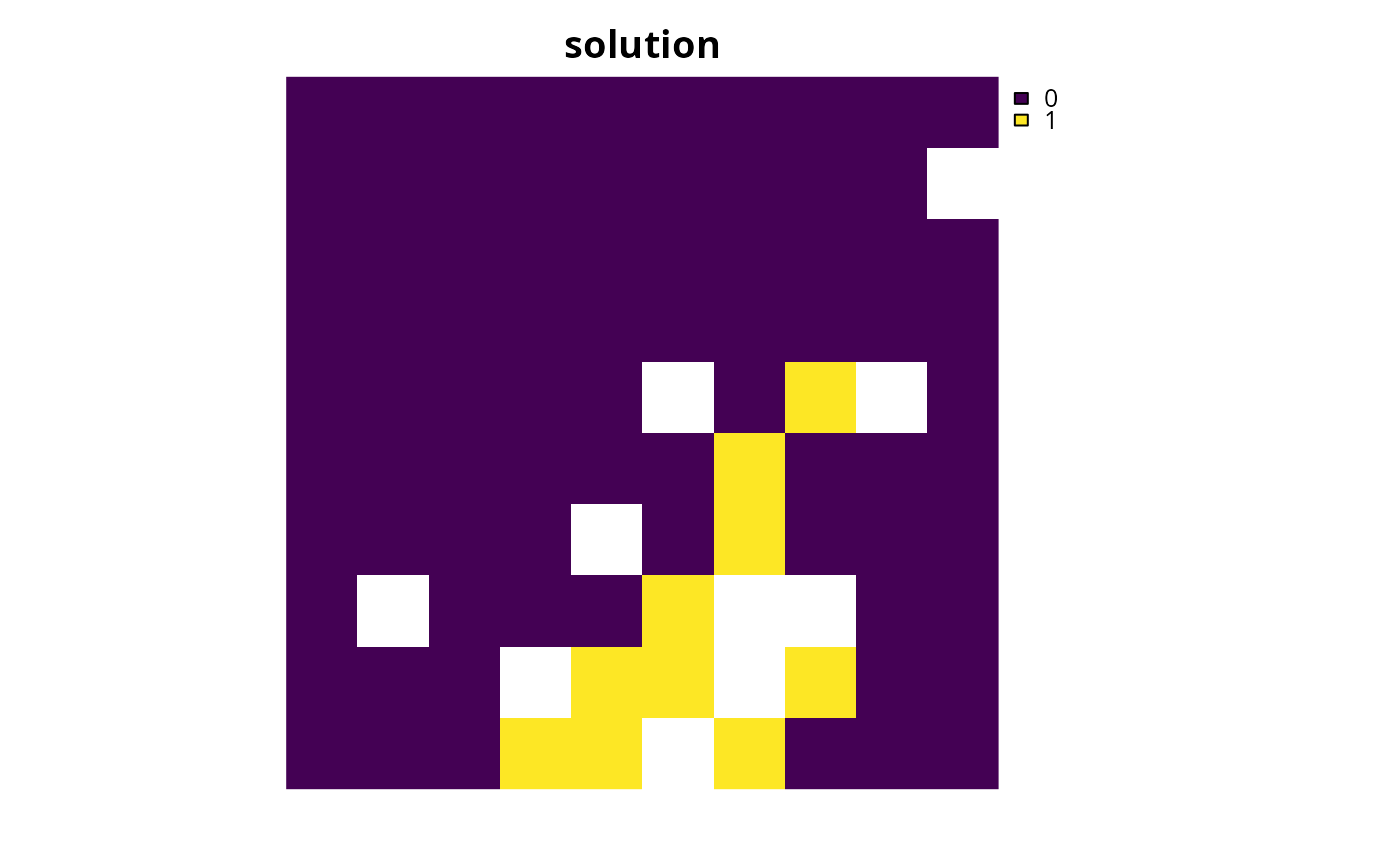# }
# create problem with targets set for only a few features
p3 <- problem(sim_pu_raster, sim_features) %>%
feature = names(sim_features)[1:3], type = "relative",
sense = ">=", target = 0.1)) %>%
# \dontrun{
# solve problem
s3 <- solve(p3)

# plot solution
plot(s3, main = "solution", axes = FALSE, box = FALSE)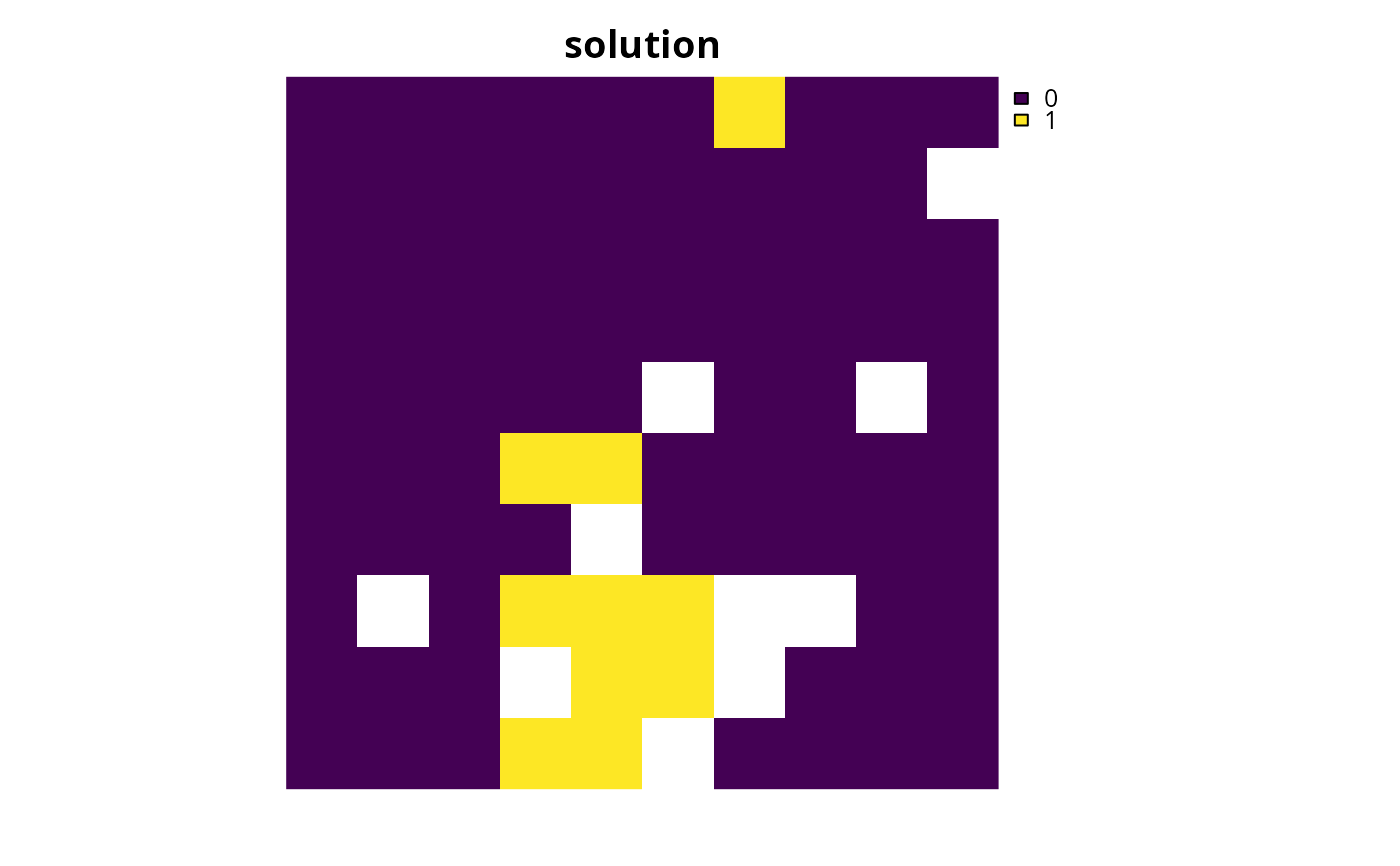# }
# create problem that aims to secure at least 10% of the habitat for one
# feature whilst ensuring that the solution does not capture more than
# 20 units habitat for different feature
# create problem with targets set for only a few features
p4 <- problem(sim_pu_raster, sim_features[[1:2]]) %>%
feature = names(sim_features)[1:2], type = "relative",
sense = c(">=", "<="), target = c(0.1, 0.2))) %>%
# \dontrun{
# solve problem
s4 <- solve(p4)

# plot solution
plot(s4, main = "solution", axes = FALSE, box = FALSE)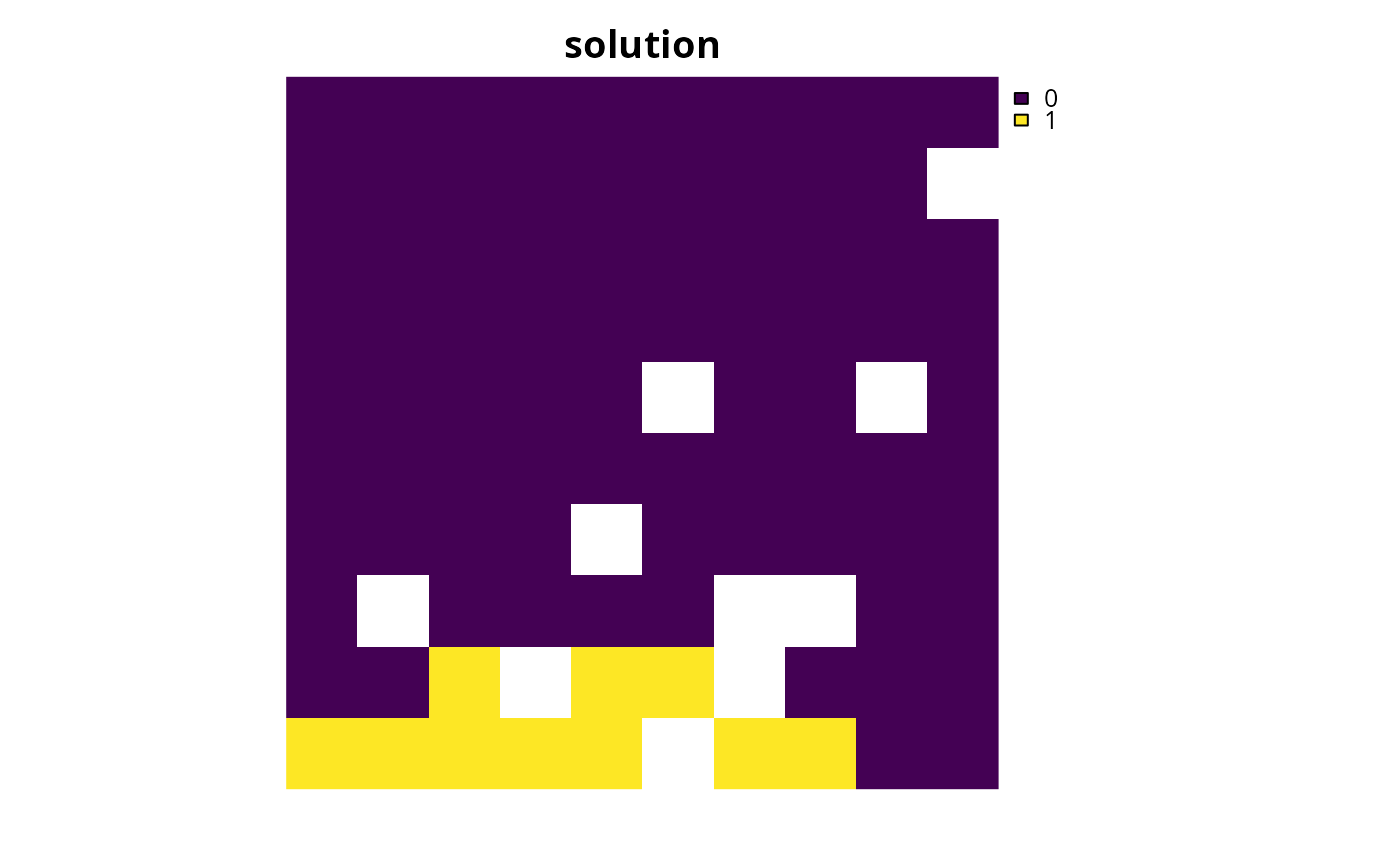# }
# create a multi-zone problem that requires a specific amount of each
# feature in each zone
targets_matrix <- matrix(rpois(15, 1), nrow = 5, ncol = 3)

p5 <- problem(sim_pu_zones_stack, sim_features_zones) %>%
# \dontrun{
# solve problem
s5 <- solve(p5)

# plot solution
plot(category_layer(s5), main = "solution", axes = FALSE, box = FALSE)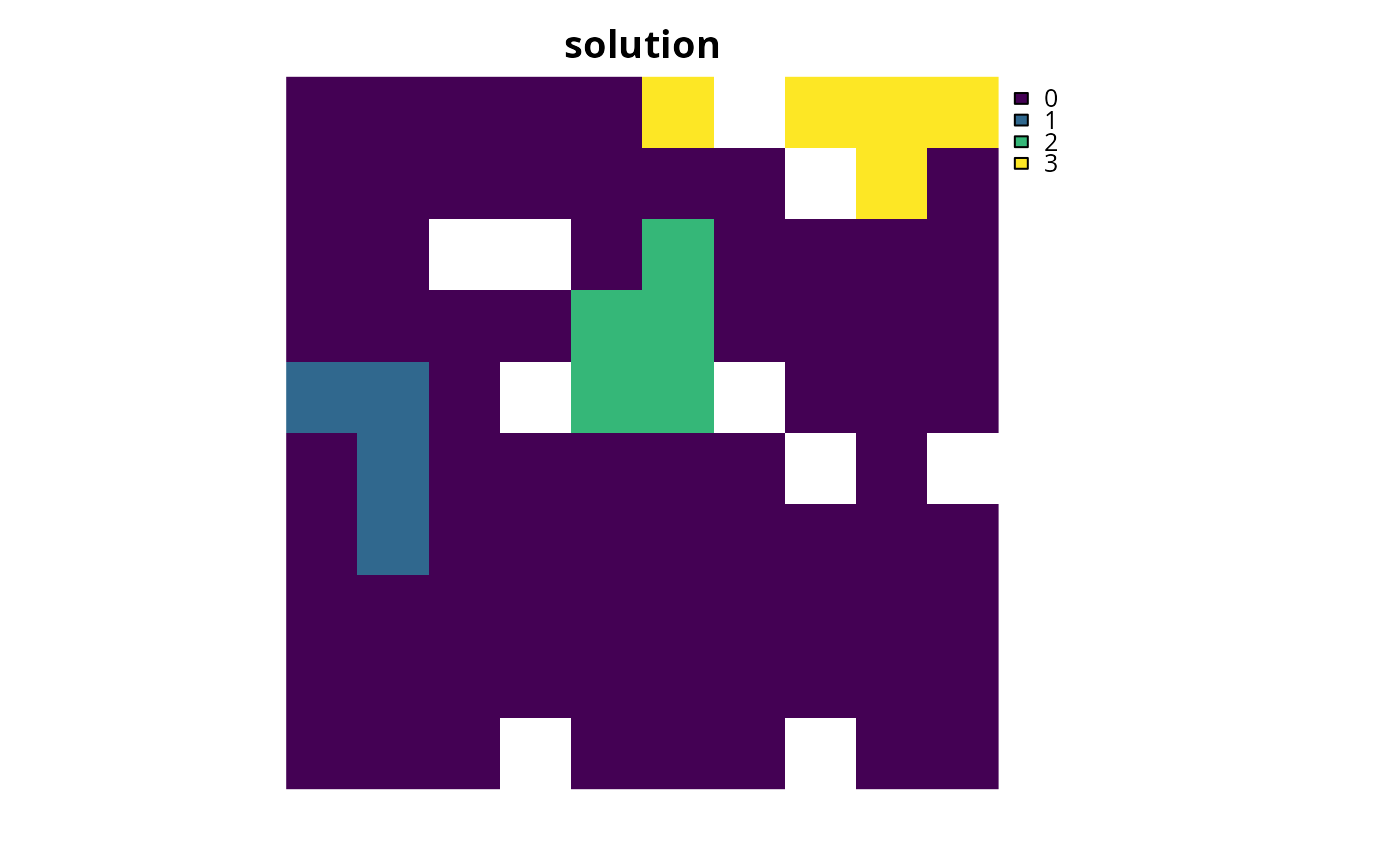# }
# create equivalent problem using add_manual_targets
targets_dataframe <- expand.grid(feature = feature_names(sim_features_zones),
zone = zone_names(sim_features_zones),
sense = ">=", type = "absolute")
targets_dataframe\$target <- c(targets_matrix)

p6 <- problem(sim_pu_zones_stack, sim_features_zones) %>%
# \dontrun{
# solve problem
s6 <- solve(p6)

# plot solution
plot(category_layer(s6), main = "solution", axes = FALSE, box = FALSE)
# }
# create a problem that requires a total of 20 units of habitat to be
# captured for two species. This can be achieved through representing
# habitat in two zones. The first zone represents a full restoration of the
# habitat and a second zone represents a partial restoration of the habitat
# Thus only half of the benefit that would have been gained from the full
# restoration is obtained when planning units are allocated a partial
# restoration

# create data
spp_zone1 <- as.list(sim_features_zones)[][[1:2]]
spp_zone2 <- spp_zone1 * 0.5
costs <- sim_pu_zones_stack[[1:2]]

# create targets
targets_dataframe2 <- tibble::tibble(
feature = names(spp_zone1), zone = list(c("z1", "z2"), c("z1", "z2")),
sense = c(">=", ">="), type = c("absolute", "absolute"),
target = c(20, 20))

# create problem
p7 <- problem(costs, zones(spp_zone1, spp_zone2,
feature_names = names(spp_zone1),
zone_names = c("z1", "z2"))) %>%